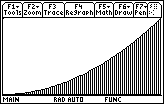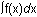Module 15 - Riemann Sums and the Definite Integral Introduction | Lesson 1 | Lesson 2 | Lesson 3 | Self-Test Introduction In this module you will learn how to estimate areas by filling the region with rectangles and adding their areas. Such sums are called Riemann sums, and you will use them to estimate the area of a region and also to find its exact area. The investigation of Riemann sums will lead to the definite integral, a basic construct of calculus. You know how to find the areas of regular geometric objects such as rectangles and triangles, but how would you find the area of a region when some of the boundaries are curves? Consider the area of the shaded region below. How would you find its area?Lesson Index: 15.1 - Right-hand Riemann Sums 15.2 - Left-hand Riemann Sums and the area Program 15.3 - The Definite Integral After completing this module, you should be able to do the following: Define right-hand and left-hand Riemann sums on the TI-89 Use Riemann sums to approximate the area under a curve Take the limit of a Riemann sum to find the exact area under a curve Visualize Riemann sums by using a program Evaluate definite integrals with the integral key on the TI-89 Evaluate definite integrals with thefeature found in the Math menu of the Graph screen on the TI-89 Next > ©Copyright 2007 All rights reserved. | Trademarks | Privacy Policy | Link Policy